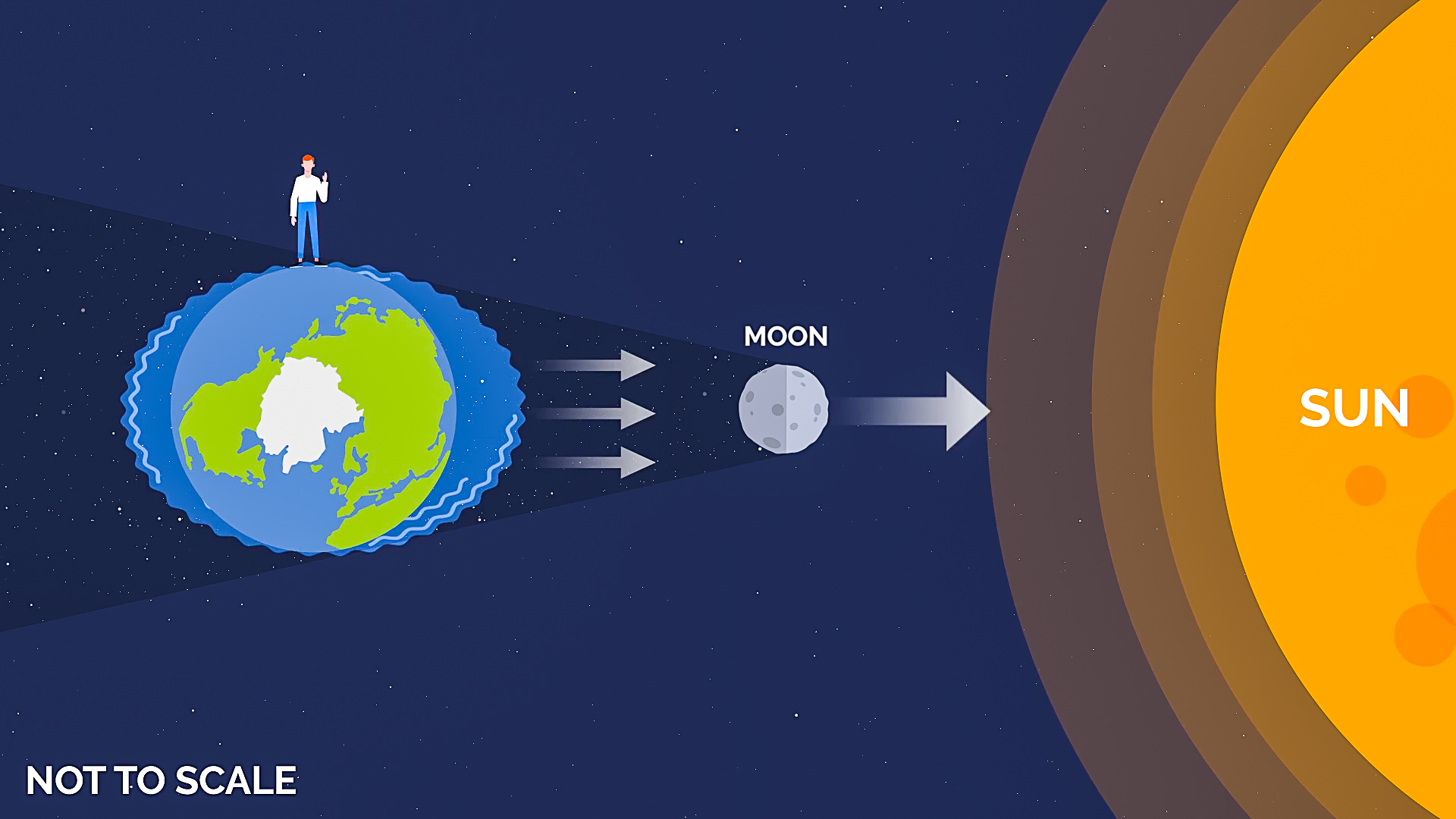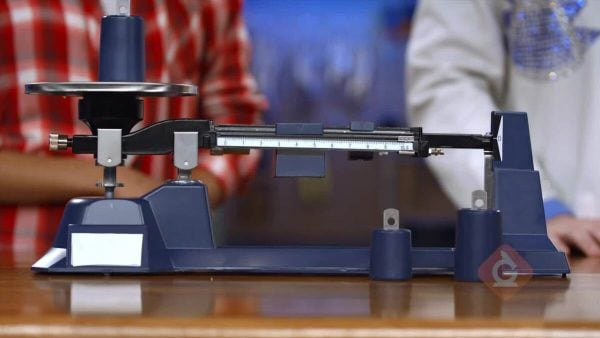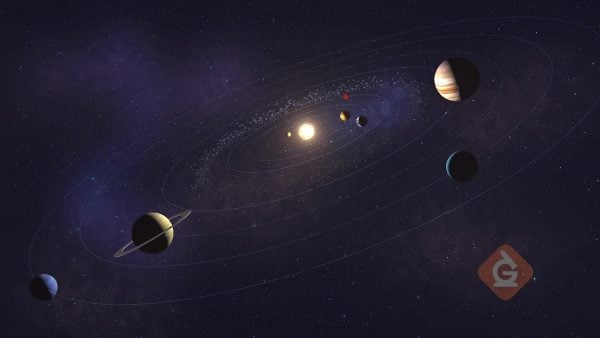Read About Gravitational Forces Between Objects | Science for Grades 6-8 [Printable]
1%
It was processed successfully!WHAT ARE GRAVITATIONAL FORCES BETWEEN OBJECTS?

On Earth, gravity gives weight to objects and pull objects toward the center of Earth. The Moon also affects this force and is responsible for the changing ocean tides. Gravitational force also occurs naturally between any objects that have mass or energy (including planets, stars, and galaxies) and pulls them together.

To better understand gravitational forces between objects…

WHAT ARE GRAVITATIONAL FORCES BETWEEN OBJECTS?. On Earth, gravity gives weight to objects and pull objects toward the center of Earth. The Moon also affects this force and is responsible for the changing ocean tides. Gravitational force also occurs naturally between any objects that have mass or energy (including planets, stars, and galaxies) and pulls them together. To better understand gravitational forces between objects…

## LET’S BREAK IT DOWN!

### Relationship Between Mass and GravityThe force of gravity depends on the size (mass) of an object. The bigger the object, the stronger the force of gravity. The force of gravity will also depend on how close or far apart one object is from another. For example, if two objects are close together, the force of gravity is greater than if they were farther apart.

Relationship Between Mass and Gravity The force of gravity depends on the size (mass) of an object. The bigger the object, the stronger the force of gravity. The force of gravity will also depend on how close or far apart one object is from another. For example, if two objects are close together, the force of gravity is greater than if they were farther apart.

### Relationship Between Mass and WeightMass and weight are different but can both be measured by standard units. Mass is the amount of matter an object contains, and mass does not change when you move an object from one place to another. Weight is the amount of force (gravity) acting on an object, and weight can change based on where an object is located.

Relationship Between Mass and Weight Mass and weight are different but can both be measured by standard units. Mass is the amount of matter an object contains, and mass does not change when you move an object from one place to another. Weight is the amount of force (gravity) acting on an object, and weight can change based on where an object is located.

### The Moon Has GravityThe Moon’s gravity pulls at Earth and causes predictable ocean tides. High tide is when the Moon’s gravitational pull is the strongest, and low tide is when the pull is the weakest. Lakes can also be affected by the Moon’s gravitational pull, but on a much smaller scale.

The Moon Has Gravity The Moon’s gravity pulls at Earth and causes predictable ocean tides. High tide is when the Moon’s gravitational pull is the strongest, and low tide is when the pull is the weakest. Lakes can also be affected by the Moon’s gravitational pull, but on a much smaller scale.

### Sir Isaac NewtonSir Isaac Newton was an Englishman who lived from 1643 to 1727. He is known in the science world for his laws of motion. Newton’s three laws of motion are commonly used in education to help students understand motion and the mathematical formulas that can be used to explain and predict motion.

Sir Isaac Newton Sir Isaac Newton was an Englishman who lived from 1643 to 1727. He is known in the science world for his laws of motion. Newton’s three laws of motion are commonly used in education to help students understand motion and the mathematical formulas that can be used to explain and predict motion.

### Careers in Science: AstronomerAstronomers are scientists who study in the science field of astronomy. Astronomers observe and study objects in space such as stars, moons, planets, asteroids and comets. Astronomers use observations and mathematical models to try to figure out what space looked like in the past and make predictions about what could happen in the future.

Careers in Science: Astronomer Astronomers are scientists who study in the science field of astronomy. Astronomers observe and study objects in space such as stars, moons, planets, asteroids and comets. Astronomers use observations and mathematical models to try to figure out what space looked like in the past and make predictions about what could happen in the future.

## GRAVITATIONAL FORCES BETWEEN OBJECTS VOCABULARY

Gravity
A force that accelerates (or pulls) an object toward the center of Earth.
Mass
The amount of matter that makes up an object. Mass does not change based on where you are.
Weight
A measurement that is taken using a scale and is determined by the pull of gravity. Weight can vary based on where you are and the pull of gravity in that area.
Resistance
A measure of a force acting in the opposite direction.
Acceleration
An increase in the rate or speed of something.
Atmosphere
The gases that surround Earth or another planet.
Every particle attracts every other particle with a force that is proportional to their masses and inversely proportional to the square of distance between them.
Any interaction that can change the motion of an object.
An Italian astronomer, physicist, and engineer. He is sometimes known as the “Father of Observational Astronomy.”
An English mathematician and physicist who is known for formulating the theory of universal gravity. Newton’s three laws are commonly studied in middle and high school.

## GRAVITATIONAL FORCES BETWEEN OBJECTS DISCUSSION QUESTIONS

### Explain one way gravity affects objects.

Gravity is the force that pull objects to the center of Earth.

### What is an example of an object that affects gravity on Earth?

The Moon affects the tides on Earth. High tide is caused by the Moon’s gravity.

### How can the force of gravity affect weight?

Gravity is not the same in all places. For example, if you were high up on a mountain, you would weigh less because the force of gravity is less.

### Use gravity to explain the relationship between Earth’s orbit around the Sun.

Although the Sun is very far way, its size keeps Earth in its orbit because of the gravitational force between the two masses.

### Explain how resistance keeps two objects of the same weight from reaching the ground at the same time.

Resistance is a force that acts in the opposite direction. If two of the same kind of umbrellas were dropped from the same height at the same time, they would hit the ground together. But if one umbrella were open, it would have more resistance and hit the ground after the one that was closed.

### Explain the difference between mass and weight.

Mass is the amount of matter that makes up an object (number of molecules an object has). Weight is measured on a scale and can change based on the force of gravity.